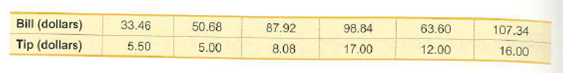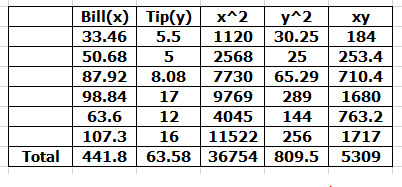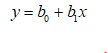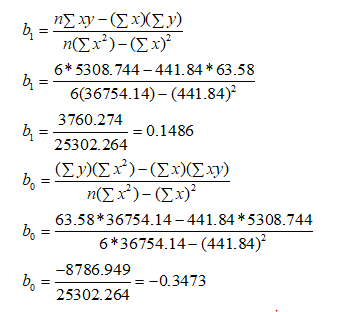# Bill (dollars)Tip (dollars)33.4650.6863.60107.3487.928.0898.845.505.0017.0012.0016.00

Question
1 views

Regression and Predictions. Exercises 13–28 use the same data sets as Exercises 13–28 in Section 10-1. In each case, find the regression equation, letting the first variable be the predictor (x) variable. Find the indicated predicted value by following the prediction procedure summarized in Figure 10-5 on page 493.

Tips Using the bill/tip data, find the best predicted tip amount for a dinner bill of \$100. What tipping rule does the regression equation suggest?help_outlineImage TranscriptioncloseBill (dollars) Tip (dollars) 33.46 50.68 63.60 107.34 87.92 8.08 98.84 5.50 5.00 17.00 12.00 16.00 fullscreen
check_circle

Step 1

Given bill tip data. Let bill be x and tip be y. The following calculations are made to find the regression line.The general regression line equation will be in form as shown belowThe values of b0 b1 are calculated as shown below...

### Want to see the full answer?

See Solution

#### Want to see this answer and more?

Solutions are written by subject experts who are available 24/7. Questions are typically answered within 1 hour.*

See Solution
*Response times may vary by subject and question.
Tagged in